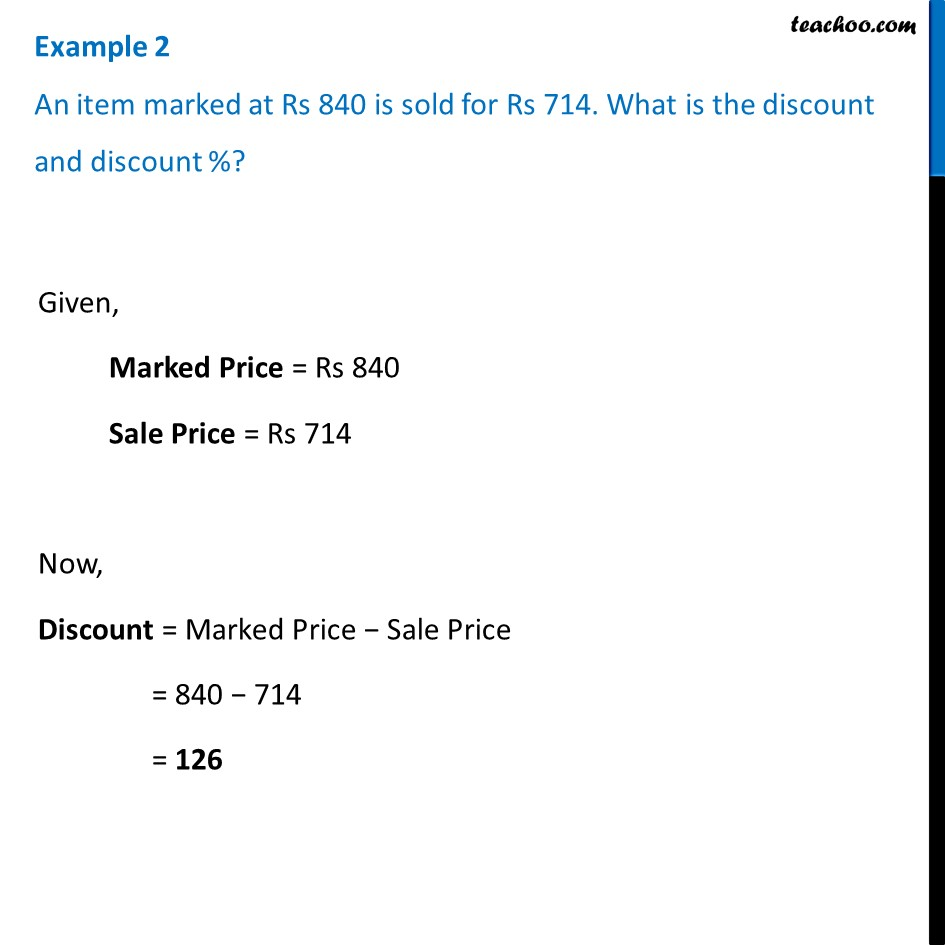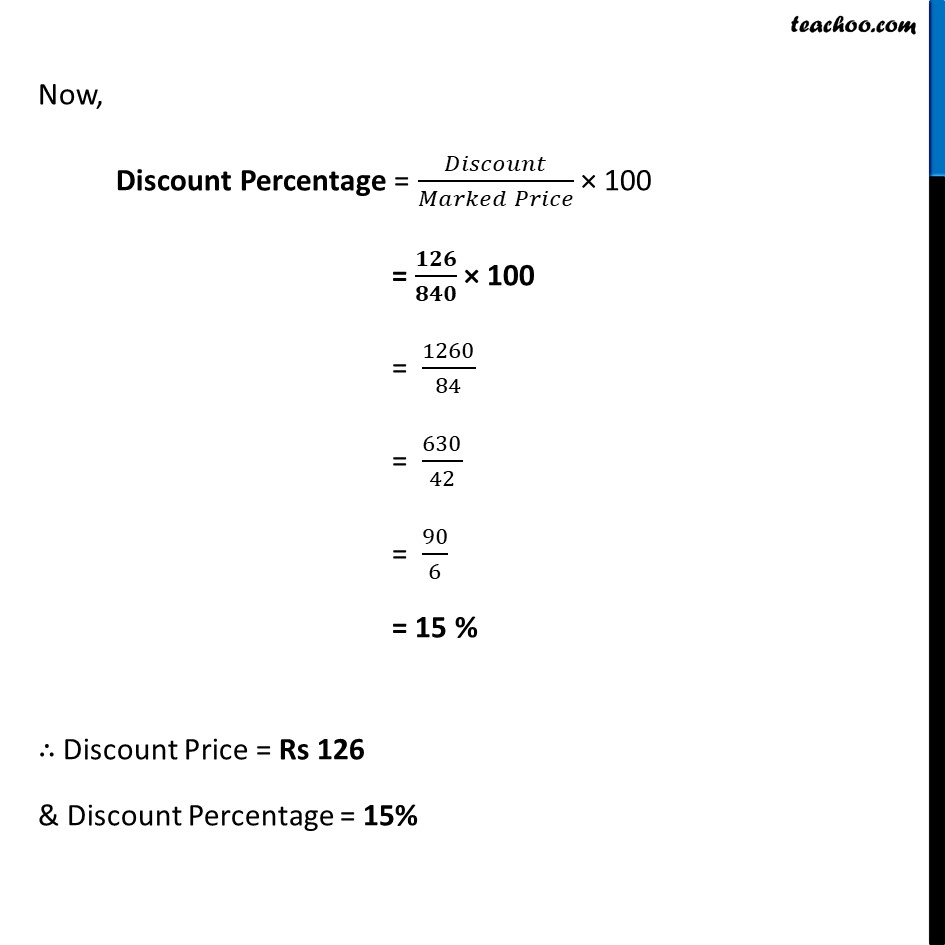Examples

Chapter 7 Class 8 Comparing Quantities
Serial order wiseLearn in your speed, with individual attention - Teachoo Maths 1-on-1 Class

### Transcript

Example 2 An item marked at Rs 840 is sold for Rs 714. What is the discount and discount %?Given, Marked Price = Rs 840 Sale Price = Rs 714 Now, Discount = Marked Price − Sale Price = 840 − 714 = 126 Now, Discount Percentage = 𝐷𝑖𝑠𝑐𝑜𝑢𝑛𝑡/(𝑀𝑎𝑟𝑘𝑒𝑑 𝑃𝑟𝑖𝑐𝑒) × 100 = 𝟏𝟐𝟔/𝟖𝟒𝟎 × 100 = 1260/84 = 630/42 = 90/6 = 15 % ∴ Discount Price = Rs 126 & Discount Percentage = 15%